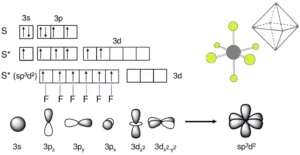# Concept of Hybridisation with Examples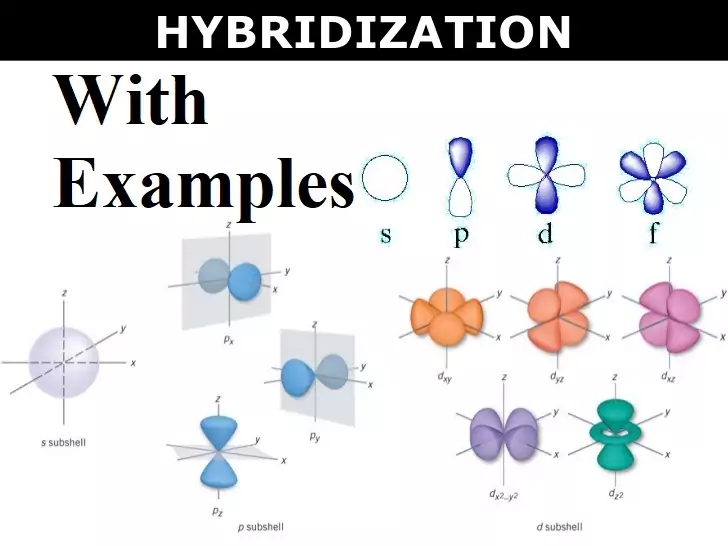Concept of Hybridisation with Examples

Concept of Hybridisation with Examples:

Hybridization: It can be defined as the process of intermixing of the orbitals of slightly different energies so as to redistribute their energies, resulting in the formation of a new set of orbitals of equivalent energies and shape.

Salient Features of hybridization:
(i) The number of hybrid orbitals is equal to the number of atomic orbitals that get hybridized.
(ii) The hybridized orbitals are always equivalent in energy and shape.
(iii) The hybrid orbitals are more effective in forming stable bonds than the pure atomic orbitals.
(iv) These hybrid orbitals are directed in space in some preferred direction to have minimum repulsion between electron pairs and thus a stable arrangement.

Conditions of hybridisation:
(i) The orbitals present in the valence shell of the atom are hybridized.
(ii) The orbitals undergoing hybridization should have almost equal energy.
(iii) Promotion of electrons is not an essential condition before hybridization.
(iv) Completely filled orbitals of valence shell can also take part in hybridization.

 Type of Hybridization Orbitals involved Arrangement of hybrid orbitals sp One s and one p Linear sp2 One s and two p Trigonal planer sp3 One s and three p Tetrahedral sp3d One s, three p, and one d Trigonal bipyramidal sp3d2 or d2sp3 One s, three p, and two d Octahedral or Square bipyramidal sp3d3 One s, three p, and three d Pentagonal bipyramidal

sp Hybridisation: This type of hybridization involves the mixing of one s and one p orbital resulting in the formation of two equivalent sp hybrid orbitals. Each sp hybrid orbitals has 50% s-character and 50% p-character. Such a molecule in which the central atom is sp-hybridized and linked directly to two other central atoms possesses linear geometry. For example

(i) BeCl2 molecule forms in the following way: In BeCl2, the central atom Be has the electronic configuration 1s22s2. In the excited state, one of the 2s electrons is promoted to the 2p level. So, the configuration becomes 1s22s12p1. Now one s-orbital and one p-orbital undergo sp hybridization to form 2 new sp hybrid orbitals. Each of these hybrid orbitals overlaps with the 2p orbitals of Cl to form 2 Be-Cl bonds. So the shape of the molecule is linear with bond angle 1800.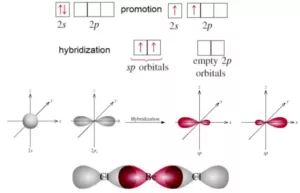(ii) Formation of Ethyne or Acetylene (C­2H2): In acetylene, each C atom undergoes sp hybridization. Out of the 2 sp hybrid orbitals, one of each C overlaps axially to form a C-C σ bond. The remaining two sp hybrid orbitals of each C overlap with the 1s orbital of H to form 2 C-H σ bonds.
Now each C atom has 2 unhybridized p-orbitals, which overlap laterally to form 2 π bonds. Thus, the molecule has a linear shape with a bond angle of 1800. Here there are 3 σ bonds and 2 π bonds in ethyne.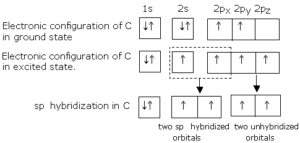sp2 Hybridisation: In this hybridization, there is the involvement of one s and two p-orbitals in order to form three equivalent sp2 hybridized orbitals. Each sp2 hybrid orbitals has 33.33% s-character and 66.66 % p-character. Such a molecule in which the central atom is sp2 hybridized and linked directly to three other central atoms possesses trigonal planar geometry. For example
(i) Formation of BCl3 molecule: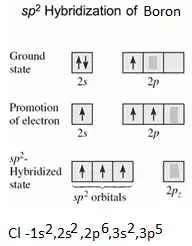sp3 Hybridisation: In this type of hybridization there is the mixing of one s-orbital and three p-orbitals of the valence shell to form four sp3 hybrid orbitals of equivalent energies and shape. There is 25% s-character and 75% p character in each sp3 hybrid orbital. The four sp3 hybrid orbitals so formed are directed towards the four corners of the tetrahedron. For Example:
(i) Formation of Methane (CH4): In CH4, the central atom C has the electronic configuration 6C – 1s22s22p2.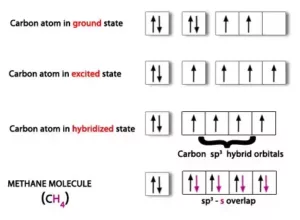(ii) Formation of Ammonia (NH3) Molecule: In NH3, the central atom N has the electronic configuration 1s22s22p3.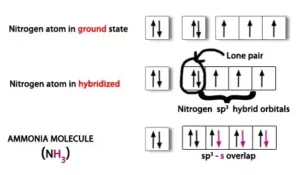The one s-orbital and three p-orbitals of N undergo sp3 hybridization to form 4 sp3 hybrid orbitals. One of these sp3 hybrid orbital is occupied by a lone pair and the other three sp3 hybrid orbitals overlap with 1s orbital of hydrogen to form 3 N-H bonds. Due to the greater repulsion between lone pair and bond pairs, the shape is distorted to pyramidal and the bond angle becomes 1070.

sp3d Hybridisation: It is the process of intermixing of one s-orbital, three p-orbitals, and one d-orbital to form five new orbitals having equivalent energy and shape. The 5 new orbitals formed are called sp3d hybrid orbitals. These are directed to the five corners of a regular trigonal bipyramid with bond angles 1200 and 900.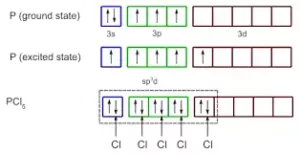In PCl5, three P-Cl bonds lie in one plane, at an angle of 1200. These three bonds are called equatorial bonds. The other two P-Cl bonds lie one above and one below this plane. They are called axial bonds. The axial bond pairs suffer more repulsion from the equatorial bond pairs. So, the axial bond length is greater than the equatorial bond length. So PCl5 is highly unstable and is very reactive.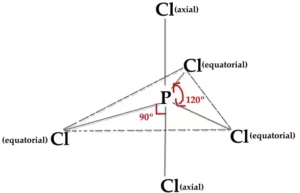sp3d2 Hybridisation: It is the process of intermixing of one s-orbital, three p-orbitals, and two d-orbitals to form six new orbitals having equivalent energy and shape. The 6 new orbitals formed are called sp3d2 hybrid orbitals. These are directed to the six corners of a regular octahedron with a bond angle 900. For example, the formation of SF6.